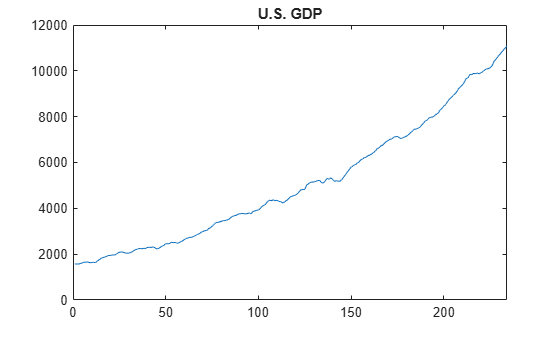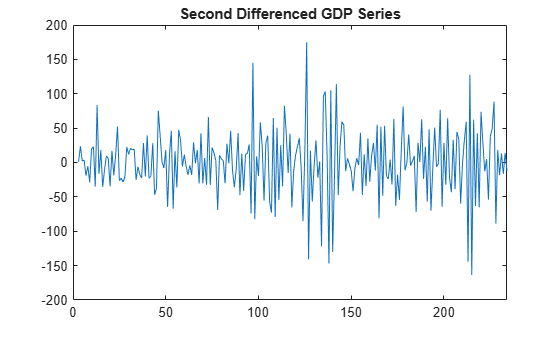Documentation

## Nonseasonal Differencing

This example shows how to take a nonseasonal difference of a time series. The time series is quarterly U.S. GDP measured from 1947 to 2005.

Load the GDP data set included with the toolbox.

```load Data_GDP Y = Data; N = length(Y); figure plot(Y) xlim([0,N]) title('U.S. GDP')```The time series has a clear upward trend.

Take a first difference of the series to remove the trend,

`$\Delta {y}_{t}=\left(1-L\right){y}_{t}={y}_{t}-{y}_{t-1}.$`

First create a differencing lag operator polynomial object, and then use it to filter the observed series.

```D1 = LagOp({1,-1},'Lags',[0,1]); dY = filter(D1,Y); figure plot(2:N,dY) xlim([0,N]) title('First Differenced GDP Series')```The series still has some remaining upward trend after taking first differences.

Take a second difference of the series,

`${\Delta }^{2}{y}_{t}=\left(1-L{\right)}^{2}{y}_{t}={y}_{t}-2{y}_{t-1}+{y}_{t-2}.$`

```D2 = D1*D1; ddY = filter(D2,Y); figure plot(3:N,ddY) xlim([0,N]) title('Second Differenced GDP Series')```The second-differenced series appears more stationary.